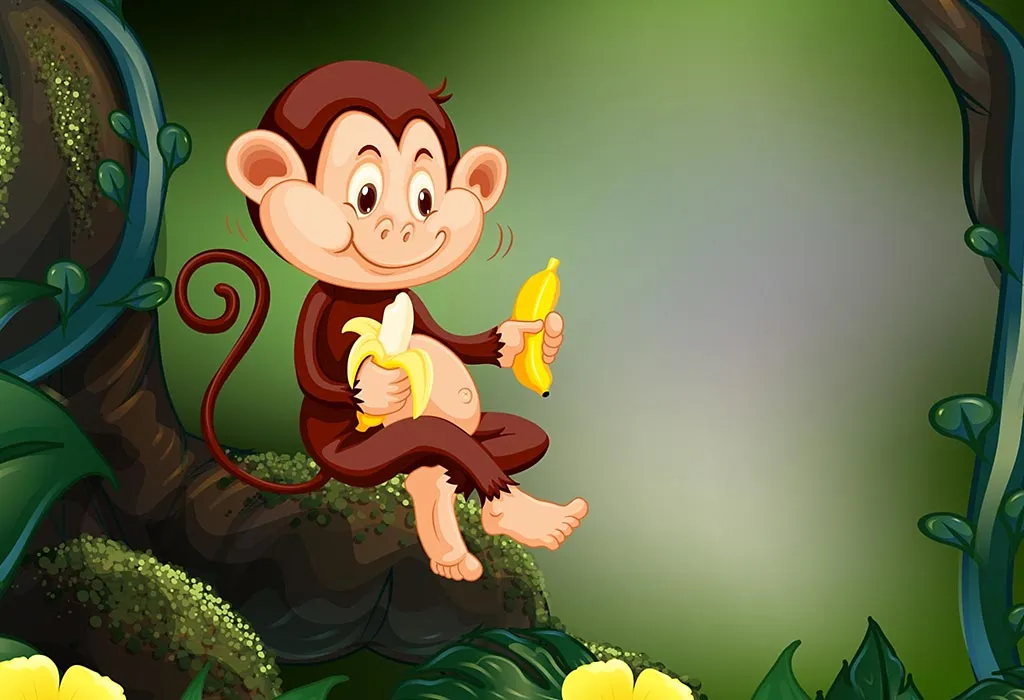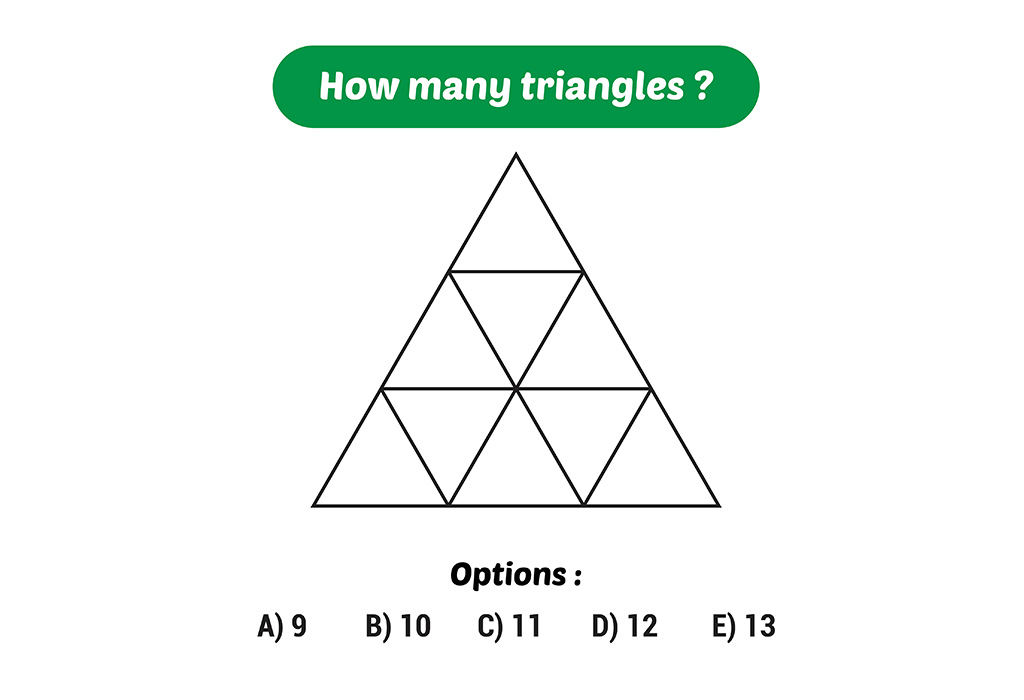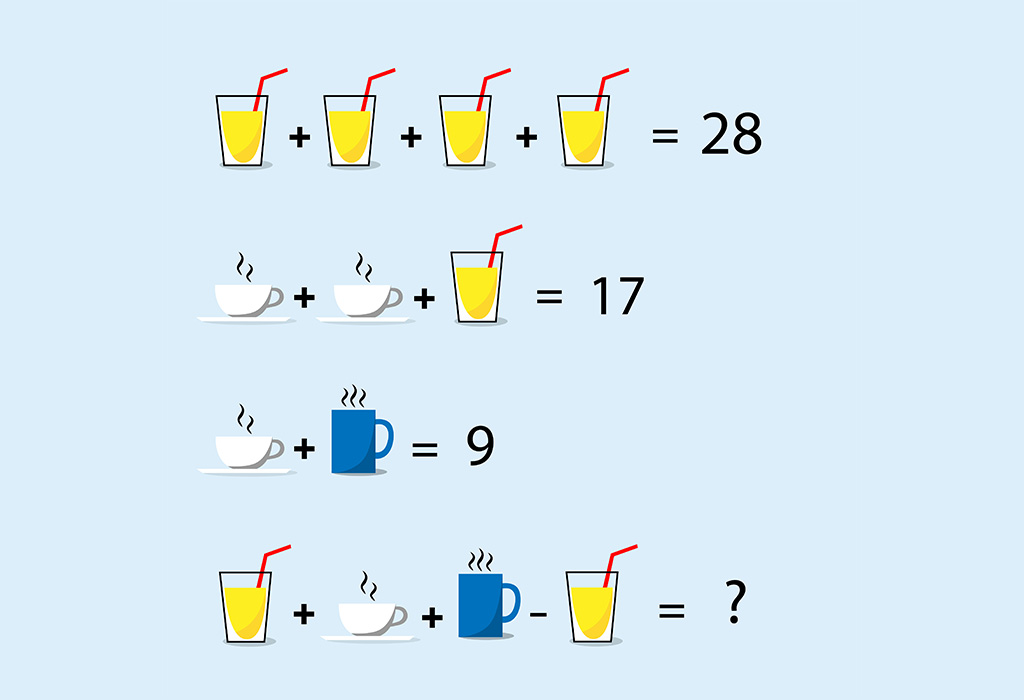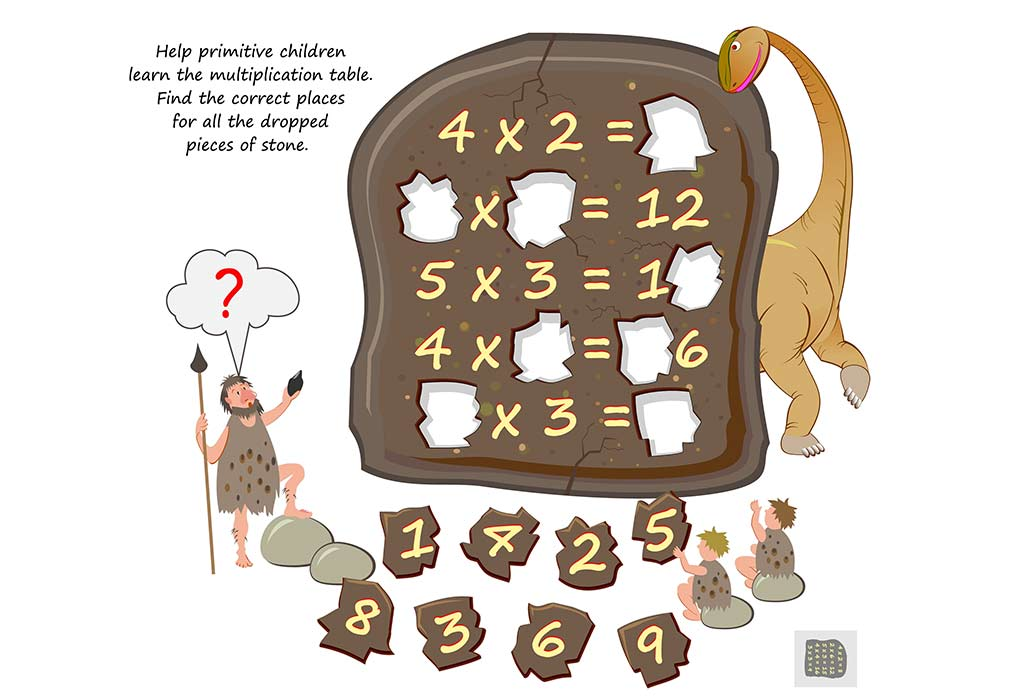25 Easy Maths Puzzles for Kids With Answers

# 25 Easy Maths Puzzles for Kids With Answers

Mathematics is a subject that children often dread or find boring. However, it does not have to be dull or intimidating. Math can be a lot of fun if taught the right way. As a parent, there’s a lot you can do at home to make this subject fun for your child. Right from riddles to puzzles to games, math no longer needs to be as hard for your child to grasp. This can be a great evening activity and help you bond with your child too. Here are simple maths puzzles with answers that will help children improve their maths skills in an interesting and enjoyable way.

## Maths Puzzles for Children

1. Ramesh turned 10 years old day before yesterday. By next year he will become 13. How is this possible?

Answer: This is one of the more amusing maths puzzles for kids age 6. With the help of math and a calendar, this puzzle can be easily explained.

For this puzzle to work, we need to assume that today is 1st January 2018. This means that Ramesh’s eleventh birthday was on 31st December 2017. Day before yesterday, he was 10 years old, today he is 11. By the end of the current year, 2018, he will be 12 years old. And so, by the next year (i.e. in 2019), he will be 13 years old.

2. What is the following number? 18, 21, 24, 27, 30, 33, 36, ______________

Answer: Here, the pattern to be observed is: every successive number is greater by 3. So the sequence will be: 18, 21, 24, 27, 30, 33, 36, 39, 42, 45, 48, and so on.

3. What is the following number? 53, 51, 49, 47, 45, 43, _______________

Answer: Here, the pattern to be observed is: every successive number is smaller by 2. So the sequence will be: 53, 51, 49, 47, 45, 43, 41, 39, 37, 35, and so on.

4. Hari had 6 siblings who were born all born 2 years apart. The youngest is Richa who is only 7 years old while Hari is the oldest. What is Hari’s age?

Answer: Richa, the youngest sibling is 7 years old. Each sibling was born 2 years apart, and there are total seven children (Hari and his 6 siblings). So Hari’s age is: 7 + 2 + 2 + 2 + 2 + 2 + 2 = 19.

5. Ravi used to live in a village that was next to the forest. One day he crossed paths with a genie. The genie was in a generous mood and granted Ravi one wish. Ravi, thinking it was a joke, wished for a 100 grams of gold. The genie agreed and behold! Ravi had a kilo of gold. Shocked and feeling upset that he asked for so little, Ravi requested the genie if he could ask for another wish. The genie agreed but only on one condition. The genie would come back next month on any date and Ravi would need to present him with gold rings. However, the gold rings had to be the same weight as the date on which he arrived. This means that if the genie came on the 20th, he would need 20 grams of gold. What did Ravi do next?

Answer: This is a challenging math riddle for kids as the date is unknown. If Ravi decided to make a different gold ring for each day, it will become problematic. This is because he only has a 100 grams of gold. So, if he made a gold ring of 31 grams,30 grams, 29 grams and 27 grams, he would run out of gold. The solution is to make just 5 rings of weight 1 gram, 2 grams, 4 grams, 8 grams and 16 grams. This can be used in combination to reach the number 31. For example:

• 24th day: 16+8
• 13th day: 8+4+1
• 7th day: 4+2+1

6. If Radha is the 50th fastest and slowest runner in her school, how many students are there in her school?

• 50th Fastest Calculation: If you count in sequence from 1, Radha comes 50.
• 50th Slowest Calculation: If you count in sequence from 99, Radha is again 50.

7. Wolves and Lambs Puzzles: Six Wolves can catch just six lambs in six minutes. So how many wolves will they need to catch 60 lambs in sixty minutes? Hint – the answer is not sixty.

Answer: 1 wolf can catch 1 lamb in six minutes. In 60 minutes, each wolf can catch 10 lambs. So, each wolf can catch ten lambs, and in sixty minutes, 6 wolves can catch 60 lambs. Another method – Six Wolves. How? 6 wolves can catch six lambs in 6 minutes. If you multiply it by ten, the same wolves can catch sixty lambs in 60 minutes.

8. How Many Cycles? This is another mathematical statement problem which can be solved using logic and arithmetic. Last weekend, Jack went to play in the park near his house. He rode the new bicycle gifted by his grandma on his birthday. After reaching the park, Jack saw that there were 14 tricycles and bicycles. If there were totally 38 wheels, how many tricycles were there in the park?

Answer: There were 10 Tricycles. There were 14 cycles in all, and all of them have at least 2 wheels. So, 14 x 2 = 28. Now there are 38 wheels totally, which means 38 – 28 = 10. So, this means there are 10 cycles with one extra wheel each, meaning 10 tricycles in all.

9. The principal is constructing a square playground for the children of her school. She wanted to fence the area with four exits around the playground so that the ground was accessible from all sides. If she used 27 poles on each side of the playground, how many poles would be needed in total?

Answer: 104 poles. The 4 corner poles will be common to two sides. Hence: (25 poles x 4 sides) + 4 corner poles= 104 poles

10. Monkey and Bananas: Assume there are five monkeys, and each monkey takes 5 minutes to eat 5 bananas. How many minutes would 4 monkeys take to eat 4 bananas? And how many monkeys would eat 30 bananas in 30 minutes?

Answer: Each monkey eats 5 bananas in 5 minutes, which means they eat 1 banana in 1 minute. So, if there are 4 monkeys and 4 bananas, they will collectively consume all 4 bananas in just 1 minute.

Similarly, if 30 bananas are being eaten in 30 minutes (i.e., 1 banana in 1 minute), this can be accomplished by a single monkey.11. What weighs more – A kilo of apples or a kilo of feathers?

Answer: They weigh the same. Each weighs exactly a kilo.

12. Using only addition, how can you add eight 8’s to get the number 1,000?

Answer: 888 + 88 + 8 + 8 + 8 =1,000

13. The ages of a father and son add up to 66. The age of the dad is the age of the son reversed. How old could they be?

Answer: There are three different answers for this: the father and son could be 51 and 15 years or 42 and 24 years old or 60 and 06 years old.

14. A genetic scientist engineered a new plant which could double its size every day. He made it grow in a circular pond with a circumference of 600 metres. If he planted it in the southern part of the pond, and the plant covered half the pond in 28 days, how many days would it take to cover the full pond?

Answer: 29 days. As mentioned in the beginning, the plant doubles in size every day.

15. If there are four apples and you take away three, how many do you have?

Answer: You took the apples so obviously you have three.

16. How many 9’s are there between 1 and 100?

17. Draw a line. Without touching it, make this line shorter.

Answer: Draw a longer line next to it. This will make the first line seem shorter.

18. There are 2 buckets, a 5 litre and a 3-litre bucket. Fill the 5-litre bucket with 4 litres of water using just these two buckets to measure.

Answer: Fill the 5-litre bucket fully. Pour it into the 3-litre bucket until it is full. Empty the 3-litre bucket. Pour the remaining 2 litres into the 3-litre bucket. Fill the 5-litre bucket completely. Finish filling the 3-litre bucket. Now the 5-litre bucket is left with exactly 4 litres of water.

19. What do the numbers 88, 96 and 11 all have in common?

Answer: They look the same upside down and right side up.

20. Two fathers and two sons go fishing. Each of them catches one fish. So why do they bring home only three fish?

Answer: The fishing group comprises a grandfather, his son, and his son’s son. So, there are just three people.

21. Peter has more than two birds at home. All the birds are parrots except for two. All of them are lovebirds except for two. All of them are pigeons, except for two. How many birds does Peter have and which ones?

Answer: This is quite an interesting math riddle as the answer is in the question itself. In the second sentence, the riddle mentions that two birds are not parrots. The third and fourth sentences mention two other birds, which is a lovebird and a pigeon. Which means, there are three birds; one parrot, one lovebird, and one pigeon.

22. There are 2 pages in a book. Each page has 3 paragraphs, and each paragraph has 5 words. How many words are there in the book?

Answer: The key to this answer is addition. Every page has 3 paragraphs with 5 words each,  so, the total number of words per page = 5 words + 5 words + 5 words = 15 words

Thus, 2 pages will have 15 + 15 words i.e. 30 words.)

## Picture Puzzles For Kids With Answers

Picture puzzles are a great way to get kids to like maths, as it stimulates vision, more than having to do mental maths. Here are easy picture puzzles for kids.

#### 1. Count the TrianglesAnswer: In this puzzle, you have to count the total number of triangles. Don’t miss out on the overlapping ones. The correct answer is option D: 13.

####Answer: The premise of this math puzzle is to first determine the value of each beverage.

• In the first line, the value of one lemonade will be 28 / 4 = 7.
• In the second line, we know that the lemonade is 7, which means two cups of tea will be 17 -7 = 10. One cup of tea will be 10 / 2 =5.
• In the third line, we know that the cup of tea is 5, so the coffee mug will be 9 – 5 = 4.
• Finally,  the result can be calculated by adding these values to the beverages: 7 + 5 + 4 – 7 = 9.

#### 3. Primitive MultiplicationAnswer: The premise of this puzzle game is to reinforce multiplication tables in kids. The answers are as follows:

• 4 X 2 = 8
• 6 X 2 = 12
• 5 X 3 = 15
• 4 X 4 = 16
• 3 X 3 = 9

## FAQs

### 1. What Are Different Types Of Math Puzzles For Children?

You can choose from a variety of math puzzles to teach your child different concepts. Here are a few of them.

• Math riddles
• Brain teasers
• Picture puzzles
• Logic puzzles
• Number puzzles
• Crossword puzzles

### 2. What are the Benefits Of Math Puzzles For Kids?

Here are some reasons why you should make use of math puzzles for your child.

• Helps kids understand topics clearly.
• Increases concentration due to interest.
• Boosts problem-solving skills.
• Helps kids stay active through the day.
• Boosts self-esteem as they are better prepared in school.
• Increases mind and body coordination.

Math can be a daunting subject for many kids but puzzle-solving will take away the fear of numbers and help your child develop an enthusiasm for numeracy. It strengthens problem-solving skills in your little one and helps him reason in all sorts of situations in a better manner. Subscribe to the child activity box and make numbers their new friend.